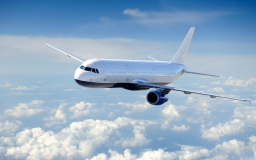# Aircraft 25161

The average climb angle of the aircraft is 11 ° 20', and its average speed is 400 km/h. How long does it take to climb to a height of 3000m?

t =  2.2899 min

### Step-by-step explanation:Did you find an error or inaccuracy? Feel free to write us. Thank you!

Tips for related online calculators
Our vector sum calculator can add two vectors given by their magnitudes and by included angle.
Do you want to convert length units?
Do you want to convert velocity (speed) units?
Do you want to convert time units like minutes to seconds?
Most natural application of trigonometry and trigonometric functions is a calculation of the triangles. Common and less common calculations of different types of triangles offers our triangle calculator. Word trigonometry comes from Greek and literally means triangle calculation.

#### Grade of the word problem:

We encourage you to watch this tutorial video on this math problem: